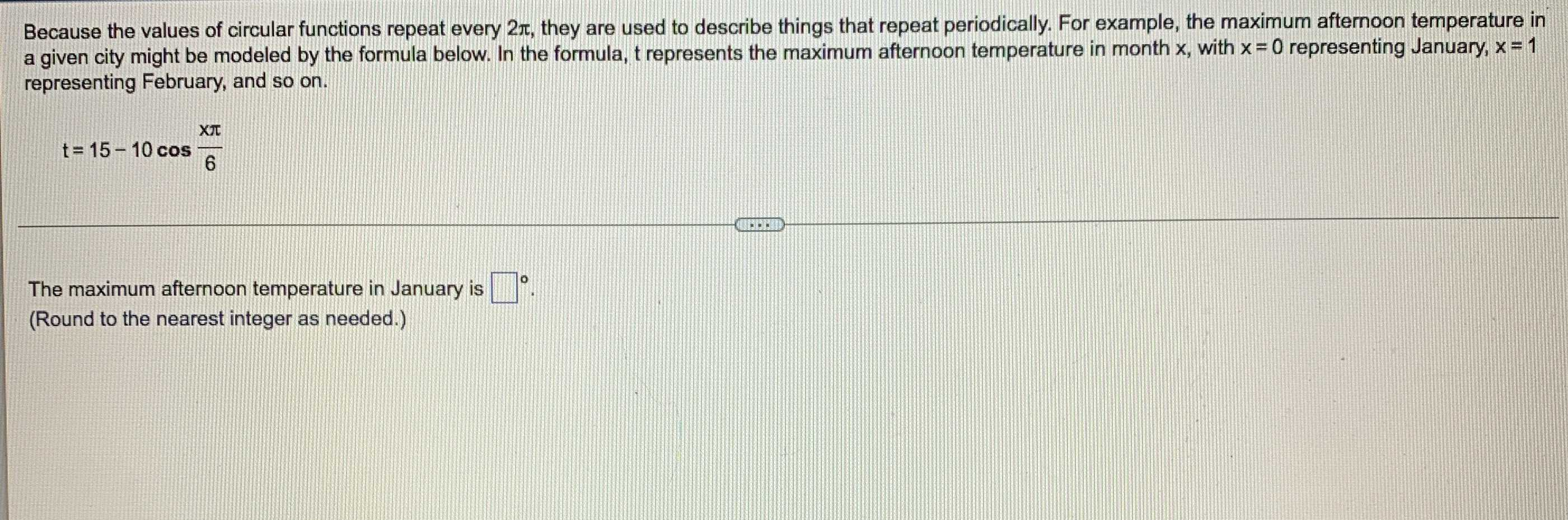### Still have math questions?

Trigonometry
QuestionBecause the values of circular functions repeat every $$2 \pi$$ , they are used to describe things that repeat periodically. For example, the maximum afternoon temperature in a given city might be modeled by the formula below. In the formula, t represents the maximum afternoon temperature in month $$x$$ , with $$x = 0$$ representing January, $$x = 1$$ representing February, and so on.

$$t = 15 - 10 \cos \frac { x \pi } { 6 }$$

The maximum afternoon temperature in January is ___. (Round to the nearest integer as needed.)